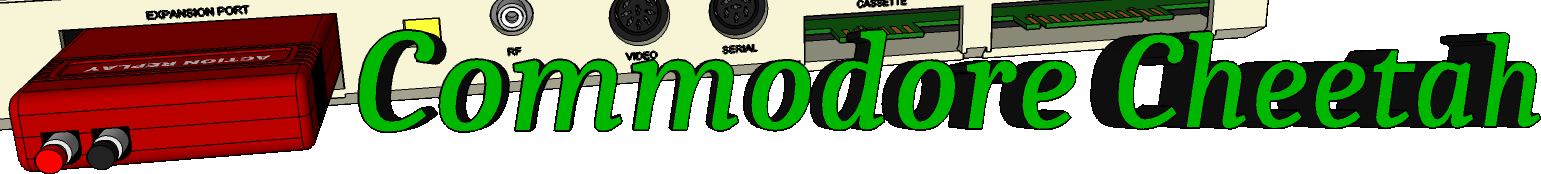Home PageeMail
email5-commodorecheetah.co.uk

Note: You will need to replace the '-' with the @ symbol.
This is to minimize spam.

 My BASIC Quick Reference Guide OR Abbreviation: TYPE: Logical Operator FORMAT: OR Action: Just as the relational operators can be used to make decisions regarding program flow, logical operators can connect two or more re-lations and return a true or false value which can then be used in a decision. When used in calculations, the logical OR gives you a bit result of I if the corresponding bit of either or both operands is 1. This will produce an integer as a result depending on the values of the operands. When used in comparisons the logical OR operator is also used to link two expressions into a single compound expression. If either of the expressions are true, the combined expression value is true (-1). In the first example below if AA is equal to BB OR if XX is 20, the expression is true. Logical operators work by converting their operands to 16-bit, signed, two's complement integers in the range of -32768 to +32767. If the operands are not in the range an error message results. Each bit of the result is determined by the corresponding bits in the two operands. EXAMPLES of OR Operator: 100 IF (AA=BB) OR (XX=20) THEN... 230 KK%=64 OR 32: PRINT KK% (You typed this with a bit value of 1000000 for 64 and 100000 for 32) 96 (The computer responded with bit value 1100000. 1100000=96.)

Commodore Cheetah made by Allen Monks, started in the year 2000.